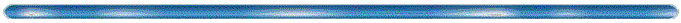EECS 723 Class HandoutsSection 2.1: The Lumped-Element Circuit Model for a Transmission Line

Section 2.3: The Terminated, Lossless Transmission Line

The Transmission Coefficient T

Section 2.4: The Smith Chart

The Complex G Plane

The Reflection Coefficient Transformation

Transformations on the Complex G Plane

Mapping Z to G

The Smith Chart

Zin Calculations using the Smith Chart

Example: The Shorted Transmission Line

Example:  Determining the length of a T. L.

Example: Admittance Calculations with the Smith Chart

Section 2.5: The Quarter-Wave Transformer

The Quarter-Wave Transformer

The Multiple Reflection Viewpoint

Section 2.6: Generator and Load Mismatches

A T. L. Connecting Source and Load

Delivered Power

Section 2.7: Lossy Transmission Lines

The Distortionless Line

Section 3.5: The Coaxial Line

Coaxial Transmission Lines

Section 3.7: Stripline

Stripline Transmission Lines

Section 3.8: Microstrip

Microstrip Transmission Lines

Section 3.11: Summary of Transmission Lines and Waveguides

A Comparison of Common Transmission Lines and Waveguides

Section 4.2: Impedance and Admittance Matricies

The Impedance Matrix

Example: Using the Impedance Matrix

Section 4.3: The Scattering Matrix

Example: The Scattering Matrix

Example: A Lossless, Reciprocal Network

Example: Determining the Scattering Matrix

Section 4.4: The Transmission Matrix

The Transmission Matrix

Section 4.5: Signal Flow Graphs

Rules for Signal Flow Graph Decomposition

Example: Decomposition of a Signal Flow Graph

Section 5.1: Matching with Lumped Elements

L-Network Analysis

Section 5.2: Single-Stub Tuning

Shunt-Stub Tuning

Series-Stub Tuning

Section 5.3: Double-Stub Tuning

Double-Stub Tuning

Section 5.4: The Quarter-Wave Transformer

The Quarter-Wave Transformer Yet Again

Section 5.5: The Theory of Small Reflections

The Multisection Transformer

Section 5.6: The Binomial Multisection Transformer

The Binomial Matching Transformer

Section 5.7: The Chebychev Matching Transformer

The Chebychev Matching Transformer

Section 5.8: Tapered Lines